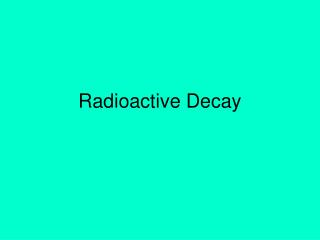DownloadDownload PresentationTélécharger la présentation- - - - - - - - - - - - - - - - - - - - - - - - - - - E N D - - - - - - - - - - - - - - - - - - - - - - - - - - -
##### Presentation Transcript

2. What do you know about Radioactivity? • All atoms are made up of __________. • What are some radioactive isotopes? • Why do some isotopes/atoms break down and decay? • What is given off as atoms break down and decay? • How can we manipulate and use this process to our advantage?

3. The Nucleus • Recall that atoms are composed of protons, neutrons, and electrons. • The nucleus of an atom contains the protons, which have a positive charge, and neutrons, which have no electric charge.

4. The Strong Force • How do you suppose protons and neutrons are held together so lightly in the nucleus? • Another force, called the strong force, causes protons and neutrons to be attracted to each other.

5. The Strong Force • Protons and neutrons have to be close together, like they are in the nucleus, to be attracted by the strong force. • The strong force is a short-range force that quickly becomes extremely weak as protons and neutrons get farther apart.

6. Attractions and Repulsion • Some atoms, such as uranium, have many protons and neutrons in their nuclei. • These nuclei are held together less tightly than nuclei containing only a few protons and neutrons.

7. Attractions and Repulsion • If a nucleus has only a few protons and neutrons, they are all close enough together to be attracted to each other by the strong force.

8. Forces in a Large Nucleus • If nuclei have many protons and neutrons, each proton or neutron is attracted to only a few neighbors by the strong force.

9. Radioactivity • When the strong force is not large enough to hold a nucleus together tightly, the nucleus can decay and give off matter and energy.Larger elements are more unstable and tend to decay. • This process of nuclear decay is called radioactivity. • All nuclei that contain more than 83 protons are radioactive. • However, many other nuclei that contain fewer than 83 protons also are radioactive.

10. Radioactivity • Almost all elements with more than 92 protons don’t exist naturally on Earth. • They have been produced only in laboratories and are called synthetic elements. • These synthetic elements are unstable, and decay soon after they are created.

11. Isotopes • Nuclei that have the same number of protons but different numbers of neutrons are called isotopes. • These two isotopes of helium each have the same number of protons, but different numbers of neutrons.

12. Stable and Unstable Nuclei • Nuclei with too many or too few neutrons compared to the number of protons are radioactive and decay too.

13. Nuclear Radiation • The three types of nuclear radiation are alpha, beta (BAY tuh), and gamma radiation. • Alpha and beta radiation are particles. Gamma radiation is an electromagnetic wave.

14. Nuclear Radiation • When an unstable nucleus decays, particles and energy called nuclear radiation are emitted from it.

15. Notice that the alpha particle and its symbol is the same as a helium nucleus, Alpha Particles • When alpha radiation occurs, an alpha particlemade of two protons and two neutrons is emitted from the decaying nucleus. • In alpha decay, two protons and two neutrons are lost from the nucleus or Helium gas is released.

16. Beta Decay • Sometimes in an unstable nucleus a neutron decays into a proton and emits an electron. • The electron is emitted from the nucleus and is called a beta particle.

17. Gamma Rays • They have no mass and no charge and travel at the speed of light. • The properties of gamma rays are summarized in the table.

18. Gamma Rays • Thick blocks of dense materials, such as lead and concrete, are required to stop gamma rays.

19. Standard: SPS3a Students will differentiate between alpha, beta decay and gamma radiation What is Nuclear Radiation?

21. Radioactive Half-Life • Some radioisotopes decay to stable atoms in less than a second. • However, the nuclei of certain radioactive isotopes require millions of years to decay. • A measure of the time required by the nuclei of an isotope to decay is called the half-life.

22. Radioactive Half-Life • The half-life of a radioactive isotope is the amount of time it takes for half the nuclei in a sample of the isotope to decay. • The nucleus left after the isotope decays is called the daughter nucleus.

23. Radioactive Half-Life • Half-lives vary widely among the radioactive isotopes. • Some geologists, biologists, and archaeologists, among others, are interested in the ages of rocks and fossils found on Earth.

24. Radioactive Dating • The number of half-lives is the amount of time that has passed since the isotope began to decay.

25. Carbon Dating • Carbon-14 has a half-life of 5,730 years and is found in molecules such as carbon dioxide. • Plants use carbon dioxide when they make food, so all plants contain carbon-14. • Only material from plants and animals that lived with the past 50,000 years contains enough carbon-14 to be measured.

26. Common Isotopes • Polonium-214 has a half life of .7 sec • Uranium-238 = 4.5 billion years • Carbon-14 = 5,730 years • Lead-212 = 10.6 hours

27. Problems • If a human body contained 50 grams of C-14 and now had only 25 g, how old is the body? • ½ of 50 is 25 so 1 half-life elapsed and that is 5,730 years

28. Problems • If the amount of C-14 is 12.5 g • 11,460 years • If the amount of C-14 is 6.25g • 17,190 years